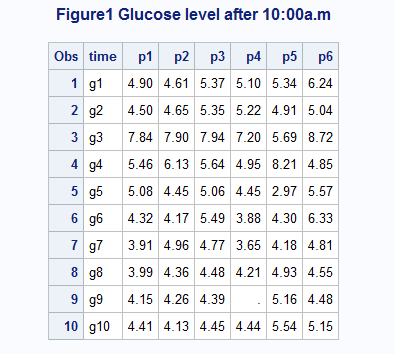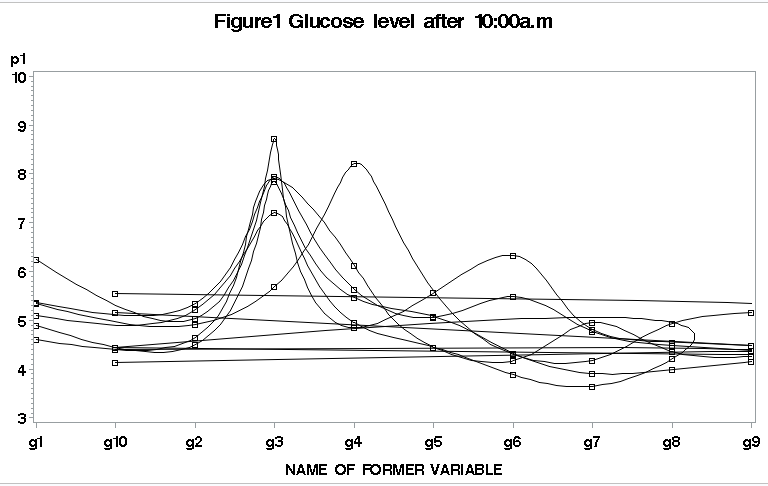## Rename name of column to certain number

Hi,

I am doing project about glucose levels after meal.

i transposed matrix

``````DATA glucose1;
set missqlucose (firstobs = 1 obs = 6);
run;

PROC TRANSPOSE data=glucose1 out=gtrans1 name=time prefix=p;
VAR g1-g10;
run;

PROC PRINT DATA= gtrans1;
run;``````

and i got that tableSo, here p1....p6 different lines on graph, where g1-g10 is X-axis and values in table is Y-axis.

Example for p1-line x=g1, y=4.90.

Moreovere, I need change g1....g10 to certain number(time):

g1=-15;
g2=0;
g3=30;
g4=60;
g5=90;
g6=120;
g7=180;
g8=240;
g9=300;
g10=360;

So when i tried plot graph

``````PROC GPLOT DATA = gtrans2;
TITLE 'Figure1 Glucose level after 10:00a.m';
PLOT
p1*time=3
p2*time=3
p3*time=3
p4*time=3
p5*time=3
p6*time=3
/overlay frame
haxis=-15 to 400
vaxis= 3 to 10 by 1
vref=0
lhref=25;

run;``````

And i got thisSo, question: How rename g1...g10 to time(certain numbers) and plot the graph for that numbers?

1 ACCEPTED SOLUTION

Accepted Solutions

## Re: Rename name of column to certain number

It looks like you reused a variable that already existed - Time - which looks like it was a character. Do you have a note in your log about conversion from numeric to character or something of the sort?

Since Time is still character it's not sorting numerically.

Create a new variable, TIME2, that is numeric and use that variable in your graphing procedure instead.

Also, you should use if/else if instead of multiple if's. It helps to speed it up, since as soon as one condition is met it stops checking th rest.

``````DATA gtrans2;
SET gtrans1;

IF (time='g1' )  THEN time2 = -15;
else IF (time='g2' )  THEN time2 = 0;
else IF (time='g3' )  THEN time2 = 30;

*rest of code follows similar format;

run;``````

4 REPLIES 4

## Re: Rename name of column to certain number

Since your time intervals aren't equal you can't apply a format, you need to recode the values. You can recode either via if/then, select, or the input function with a custom format.

I would suggest an if/then or a select if your just learning SAS.

Here's some examples of recording using if/then

http://www.ats.ucla.edu/stat/sas/modules/vars.htm

And here's an example of Select (and another if/then)

http://www.ats.ucla.edu/stat/sas/library/SASTranMan_os.html#SELECT%20case%20distinction

## Re: Rename name of column to certain number

``````DATA gtrans2;
SET gtrans1;

IF (time='g1' )  THEN time = -15;
IF (time='g2' )  THEN time = 0;
IF (time='g3' )  THEN time = 30;
IF (time='g4' )  THEN time = 60;
IF (time='g5' )  THEN time = 90;
IF (time='g6' )  THEN time = 120;
IF (time='g7' )  THEN time = 180;
IF (time='g8' )  THEN time = 240;
IF (time='g9' )  THEN time = 300;
IF (time='g10')  THEN time = 360;
run;``````

but now, when i use gplot

``````PROC GPLOT DATA = gtrans2 sort by time;
TITLE 'Figure1 Glucose level after 10:00a.m';
PLOT
p1*time=3
p2*time=3
p3*time=3
p4*time=3
p5*time=3
p6*time=3
/overlay frame
haxis=-15 to 400 by 15
vaxis= 3 to 10 by 1
vref=0
lhref=25;

run;``````

i got this;so, i got following problems:

1) They have same length interval (but should be different

2) not sorted

3) i need interval 15, so: -15 0 15 30 45 ......Although i have for -15, 0,    but no data for 15, 'cause next one is 30. So line should be     from 0 to 30

Thank you

## Re: Rename name of column to certain number

It looks like you reused a variable that already existed - Time - which looks like it was a character. Do you have a note in your log about conversion from numeric to character or something of the sort?

Since Time is still character it's not sorting numerically.

Create a new variable, TIME2, that is numeric and use that variable in your graphing procedure instead.

Also, you should use if/else if instead of multiple if's. It helps to speed it up, since as soon as one condition is met it stops checking th rest.

``````DATA gtrans2;
SET gtrans1;

IF (time='g1' )  THEN time2 = -15;
else IF (time='g2' )  THEN time2 = 0;
else IF (time='g3' )  THEN time2 = 30;

*rest of code follows similar format;

run;``````

## Re: Rename name of column to certain number

Thank you. It works!!!!
Discussion stats
• 4 replies
• 1002 views
• 1 like
• 2 in conversation